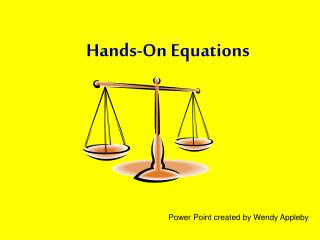DownloadDownload PresentationHands-On Equations

# Hands-On Equations

Download Presentation## Hands-On Equations

- - - - - - - - - - - - - - - - - - - - - - - - - - - E N D - - - - - - - - - - - - - - - - - - - - - - - - - - -
##### Presentation Transcript

1. Hands-On Equations Power Point created by Wendy Appleby

2. Hands-On Equations Is Algebra For Middle School Students

3. Level ILesson #1 through #7 Solve Such Equations As: 2x + x + x = 2x + 10 And 2(x + 4) + x = x + 16

4. Lesson 1Solve by Trial and Error 3 3 4 4 Needs to be The same number 8 6 6 6 2 8

5. Lesson 2Solve by Trial & Error Pawn has a Special Name “x” 2x + x = x + 8 Students are given their Student Kits Use trial and error to solve Practice: 3x + 1 = x + 7 4 4 4 4 Do Check 12 = 12 8 Do Check 10 = 10 3 3 3 3 1 7

6. Lesson 3“Legal Move” Legal Move- A move which leaves a balanced system in balance. 4x + 2 = 3x + 9 x = 7 When doing a check make sure you put the answer into the original problem. 2 9 7 7 7 7 7 7 7 2 9 30 = 30

7. Lesson 3 Practice Use original problem for check. 5x + 2 = 2x + 14 2x + x + 4 = 4x + 1 X = 4 check 22 = 22 X = 3 check 13 = 13

8. Lesson 4Legal Move with # Cube Legal Move- Subtract the same number-cube value from each side. 2x + 4 = 10 Put answer in original problem and make sure the sides balances. 4 6 4 X = 3 3 3 4 6 4 10 = 10

9. Lesson 4Using Both Legal Moves Use original problem for check. 4x + 5 = 2x + 13 Practice: 2x + x + x + 2 = 2x + 10 8 5 5 X = 4 Check 21 = 21 X = 4 Check 18 = 18

10. Lesson 5Subtraction by the Removal of Pawns Use original problem for check. 5x – 3x + 2 = x + 5 Practice: 2x + x – x + 1 = x + 9 2 5 3 X = 3 Check 8 = 8 Take three off X = 8 Check 17 = 17

11. Lesson 6Distributive Property Use original problem for check. 2(x + 3) = x + 8 Practice: 2(2x + 1) = 18 3 3 8 2 X = 2 Check 10 = 10 Double what’s inside the parenthesis X = 4 Check 18 = 18

12. Lesson 7Pictorial Representation • Students transfer their hands-on learning with the game pieces to a pictorial notation using paper and pencil. 4x + 3 = 3x + 9 x x x x x x x Use original problem for check. 6 3 9 X = 6 Check 27 = 27

13. Lesson 7Pictorial Notation Use original problem for check. • 2(x + 4) + x = x + 16 X = 4 Check 20 = 20 4 X X 8 4 16 X X

14. End of Level 1 Questions